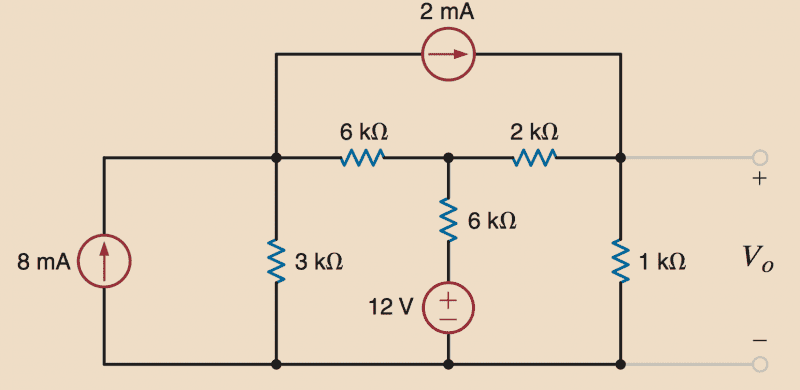# Nodal Analysis

Homework Helper

## Homework Statement

Use nodal analysis to find ##V_o##.##\sum I = 0##
##I = V/R##

## The Attempt at a Solution

So the book started throwing voltage sources into nodal analysis and I'm a bit unsure about this problem. The answer is listed as ##V_o = 3.89 V##.

All they have taught so far is to apply KCL to find the node voltages. Also, if a voltage source is connected between ground and a node, then the voltage at that node is equal to the voltage of the source.

This leads me to an important question about the problem at hand. I believe ground is located directly beneath the ##12 V## independent voltage. So couldn't I say directly above the voltage source and below the resistor is some ##V_x = 12 V##?

Finding the voltage ##V_2## across the middle branch has proven to be troublesome.

## Answers and Replies

You do know how to apply KCL at nodes right. I am following these notations as you have not marked node voltages in your figure.

#### Attachments

•STEMucator
Since we know the voltage below 6kohm resistor is 12 V and at the node we have considered V2 in the middle branch (that is V2 is the voltage across the whole middle branch).
Thus we can see that voltage drop across 6kohm resistor is (V2 -12 ),now as we know the voltage drop we can calculate the current through 6kohm resistor by using ohm's law (Voltage drop/current).

Homework Helper
You do know how to apply KCL at nodes right. I am following these notations as you have not marked node voltages in your figure.

Yes I know how to use KCL and I had those equations a while ago.

Since we know the voltage below 6kohm resistor is 12 V and at the node we have considered V2 in the middle branch (that is V2 is the voltage across the whole middle branch).
Thus we can see that voltage drop across 6kohm resistor is (V2 -12 ),now as we know the voltage drop we can calculate the current through 6kohm resistor by using ohm's law (Voltage drop/current).

This post was helpful I was unsure about how to treat the ##12 V## source. I see now that we can use the difference between ##V_2## and the source to find the current across that branch.

The rest is easy.

•lazyaditya